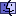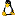Increasing Script Performance, Part IWhy Some Things Are Faster Than Others - by Wil Dijkstra

All those guys that migrated from good old HyperCard to MC, were delighted by the speed enhancement. Nevertheless, in a number of cases, MC is slow compared to languages like Pascal or C. Scott Raney gives some good advice about how to speed up your scripts. However, there are some of less known tricks that can speed up your scripts amazingly. Suppose you want to create a list consisting of a number of lines with something put into each line. For the sake of simplicity, let us create a list of 10000 random numbers between 1 and 1000. If your script looks like this:

script A:

```repeat with i = 1 to 10000
put random (1000) into line i of randList
end repeat
```
and you are eager to know how to write a script that executes more then ten times as fast, you should read on! But first some questions. Do you think that the same script, but now generating random numbers between 1 and 1000000 (one million) takes more time? If your answer is yes, youre right. It will take about twice as much time. Do you think thats because generating a random number between 1 and 1000000 takes more time than generating a random number between 1 and 1000? If your answer is yes, youre wrong. It takes about the same amount of time. To write scripts that executes as fast as possible, you should know something about how a computer (or MetaCard) works, how data are stored, etc. Im going to tell you that in a very, very simplified way. But first, the fast script:

script B:

```repeat with i = 1 to 10000
put random (1000) & cr after randList
end repeat
delete last char of theList
```
Script B yields exactly the same result as script A, but is more then ten times as fast. If we wanted to generate random numbers between 1 and 1000000, it is even more then 20 times as fast.

Here is the reason why:

Data are stored in memory. Each piece of data has a particular length. For example, in script A, the 75th call to random (1000) may yield 234, which is three characters long. This piece of data had to be put after the CR (carriage return) at the end of line number 74. But where in memory is that? The computer can only figure that out by counting the number of CRs from the start of randList. And that takes time. And each call to random (1000) the computer (the poor thing) starts counting the CRs again.

Script B does not have this drawback. The computer does not have to bother about counting CRs, but can just put the result of random (1000) after the last char of randList. In script B a CR is placed after this line, to warrant that each new result of random (1000) appears on a new line. Finally, the last cr is deleted, to ensure that the results of script A and B are exactly the same. To count the number of CRs in script A, the computer has to walk through all characters of randList and to decide whether or not the character is a CR.

If we generated random numbers between 1 and 1000000, the computer has to walk through about twice (actually, less than twice, because also the CRs are characters) as much characters than in case of generating random numbers between 1 and 1000. Hence generating random numbers between 1 and 1000000 with script A takes about twice as much time than generating random numbers between 1 and 1000 with script A. In script B there is no difference of course.

If you understand the principle, you can easily imagine that the speed increase will become negligible if the number of characters on a line is very small, but very large if the mean number of characters on each line as large.

Moreover, you will also understand why using array variables is so fast. Array variables are indexed. If the variable AR is an array variable, AR directly points to the correct position in memory. Hence, a statement like `get AR ` is very fast. On the other hand, the statement `get line 345 of randList` is much slower: again, the computer has to count 344 CRs, walking through the data of randlist.

Similarly, if you want to perfom some action on each number in randList using the script:

script C:

```repeat for each line randNumber in randList
put randNumber into temp
-- do something
end repeat
```
is much faster then

script D:

```repeat with i = 1 to the number of lines of randList
put line i of randList into temp
-- do something with temp
end repeat
```
Why? In script D the computer has to count CRs to get the is line. In script D, the computer remembers where it is, getting lines; it just proceeds through the lines and doesnt count CRs. Moreover, of course, the statement put randNumber into temp is superfluous.

A strategy that can give good results, is:

1. Put a list into an array, using the repeat for each approach. Note that you can also use items, or words.
2. Perform actions on the content of the array.
3. Put it back into the list, e.g. to display it in a field. This approach is especially useful if you want to change the contents of the list, because you cannot use repeat for each and at the same time change the contents (you should now understand why).
Happy programming, Wil Dijkstra

Posted on 4/1/03 by Will Dijkstra to the MetaCard list   (See the complete post/thread)

Some Othing Things to Remember - by Xavier Bury

Another 2 things to consider in your loops:

1. `set cursor to busy` will slow down scripts considerably.
2. If you have a "progress" bar, dont change it at every loop, do so for each percent change or at every 100th... this will also save major time...
for example:
```repeat with x = 1 to 100000
if char -3 of x is not oldx then
set cursor to busy
showstatus
put char -3 of x into oldx
end if
```
So remember to avoid screen updates...

Another consideration is

```if x is in "picasso" or x is in "miro" then...
is slower than
if x is in "picassomiro" then
```
But to avoid mistakes or substring-matching errors,
```if x is among the items of "picasso,miro" then...
```
Enjoy more speed! Xavier

Posted on 3/31/03 by Xavier Bury to the MetaCard list   (See the complete post/thread)